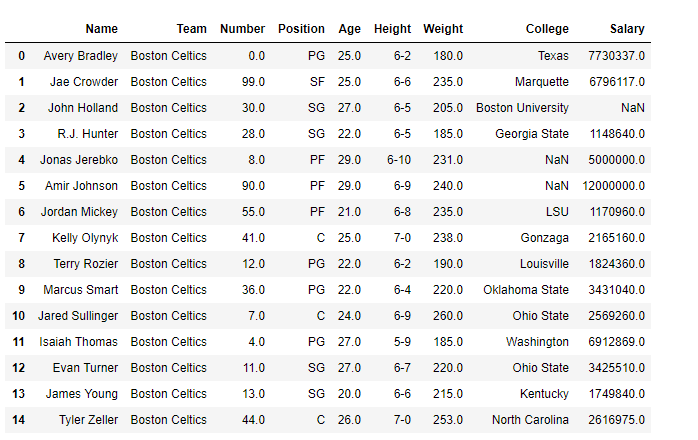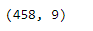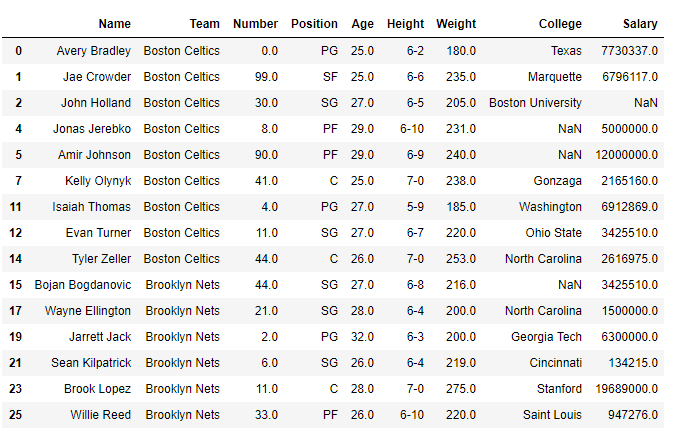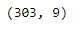# Drop rows from the dataframe based on certain condition applied on a column

## Drop rows from the dataframe based on certain condition applied on a column

Pandas provides a rich collection of functions to perform data analysis in Python. While performing data analysis, quite often we require to filter the data to remove unnecessary rows or columns.

We have already discussed earlier how to drop rows or columns based on their labels. However, in this post we are going to discuss several approaches on how to drop rows from the dataframe based on certain condition applied on a column. Retain all those rows for which the applied condition on the given column evaluates to `True`.

You are given the “nba.csv” dataset. Drop all the players from the dataset whose age is below 25 years.

Solution #1 : We will use vectorization to filter out such rows from the dataset which satisfy the applied condition.

 `# importing pandas as pd` `import` `pandas as pd` ` ` `# Read the csv file and construct the ` `# dataframe` `df ``=` `pd.read_csv(``'nba.csv'``)` ` ` `# Visualize the dataframe` `print``(df.head(``15``)` ` ` `# Print the shape of the dataframe` `print``(df.shape)`

Output :In this dataframe, currently, we are having 458 rows and 9 columns. Let’s use vectorization operation to filter out all those rows which satisfy the given condition.

 `# Filter all rows for which the player's` `# age is greater than or equal to 25` `df_filtered ``=` `df[df[``'Age'``] >``=` `25``]` ` ` `# Print the new dataframe` `print``(df_filtered.head(``15``)` ` ` `# Print the shape of the dataframe` `print``(df_filtered.shape)`

Output :As we can see in the output, the returned dataframe only contains those players whose age is greater than or equal to 25 years.

Solution #2 : We can use the `DataFrame.drop()` function to drop such rows which does not satisfy the given condition.

 `# importing pandas as pd` `import` `pandas as pd` ` ` `# Read the csv file and construct the ` `# dataframe` `df ``=` `pd.read_csv(``'nba.csv'``)` ` ` `# First filter out those rows which` `# does not contain any data` `df ``=` `df.dropna(how ``=` `'all'``)` ` ` `# Filter all rows for which the player's` `# age is greater than or equal to 25` `df.drop(df[df[``'Age'``] < ``25``].index, inplace ``=` `True``)` ` ` `# Print the modified dataframe` `print``(df.head(``15``))` ` ` `# Print the shape of the dataframe` `print``(df.shape)`

Output :As we can see in the output, we have successfully dropped all those rows which do not satisfy the given condition applied to the ‘Age’ column.

Last Updated on October 24, 2021 by admin

## Change Data Type for one or more columns in Pandas DataframeChange Data Type for one or more columns in Pandas Dataframe

Change Data Type for one or more columns in Pandas Dataframe   Let’s see the

## Python | Pandas dataframe.mul()Python | Pandas dataframe.mul()

Python | Pandas dataframe.mul() Python is a great language for doing data analysis, primarily because

## Apply function to every row in a Pandas DataFrameApply function to every row in a Pandas DataFrame

Apply function to every row in a Pandas DataFrame   Python is a great language

## Create a Pandas Series from arrayCreate a Pandas Series from array

Create a Pandas Series from array Pandas Series is a one-dimensional labelled array capable of holding

## Join two text columns into a single column in PandasJoin two text columns into a single column in Pandas

Join two text columns into a single column in Pandas Let’s see the different methods

## Pandas dataframe.append()Pandas dataframe.append()

Python is a great language for doing data analysis, primarily because of the fantastic ecosystem

## Creating a Pandas Series from DictionaryCreating a Pandas Series from Dictionary

Creating a Pandas Series from Dictionary A Series is a one-dimensional labelled array capable of